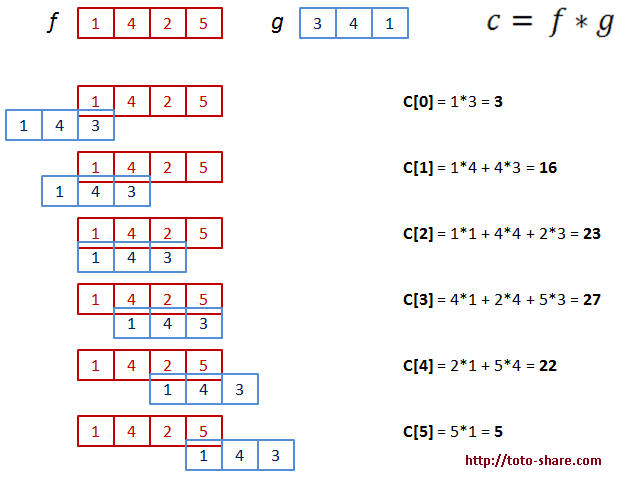# C/C++ : Convolution Source Code

In mathematics and, in particular, functional analysis, convolution is a mathematical operation on two functions f and g, producing a third function that is typically viewed as a modified version of one of the original functions (from wikipedia.com).Convolution of two square pulses: the resulting waveform is a triangular pulse. One of the functions (in this case g) is first reflected about τ = 0 and then offset by t, making it g(t − τ). The area under the resulting product gives the convolution at t. The horizontal axis is τ for f and g, and t for.

I get this picture convolution ilustration from wikipedia. We can ilustrated the convolution process with number. Please check the picture below, I try to create a convolution ilustration. So, we can create C/C++ : Convolution Source Code from this convolution ilustration :Convolution Source Code Ilustration

This is a simple convolution ilustration with input two vector. From this ilustration, we can create a C/C++ : Convolution Source Code. This is a function to compute convolution 1D with C/C++ :

we can running this code with command :

./convolution length_vect_1 length_vect_2

example :

./convolution 4 6

This is sample output from C/C++ : Convolution Source Code:

You can download the complete C/C++ : Convolution Source Code with testing program at here.

Source :
http://en.wikipedia.org/wiki/Convolution

## 4 thoughts on “C/C++ : Convolution Source Code”

1.MIMO says:

thanks for this great function in matlab 😀

2.Eder says:

Hello,
I’m working with the ambiguity function, this is used in signals…. I have to implement the ambiguity function in C language, but is very difficult to me. I have some implementations in Matlab but I need it in C.
I see that you have a C code of convolution, that is more or less that I have to implement, I would like if you can share your C source code, I will be very gratefull with you.
My email eticoa@hotmail.com
pd. Sorry for my english…

•totosugito says:

Hi, Please check the below from my post. I am attach a complete source code for this convolution problem.
Thank you

3. […] have created a code to compute convolution from my previous post : C/C++ : Convolution Source Code. That code compute convolution from two vector. Matlab have parameter to get size of the ouput data […]

This site uses Akismet to reduce spam. Learn how your comment data is processed.